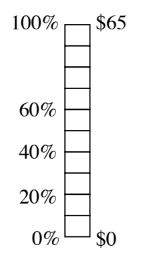### Home > MC1 > Chapter 7 > Lesson 7.3.2 > Problem7-114

7-114.

Mary is taking advantage of the $20\%$ off sale at Cassie's Cashew Shoppe and wants to figure out how much she will save on a purchase of $\65$. She drew the percent ruler shown above right. Copy the ruler on your paper and help her figure out what $20\%$ of $\65$ is.$20\%$ is one-fifth of a whole. If you divide $65$ into five parts, how much money is in each part?

$10\%$ of $65$ is $6.50$. What is $20\%$ of $65$?